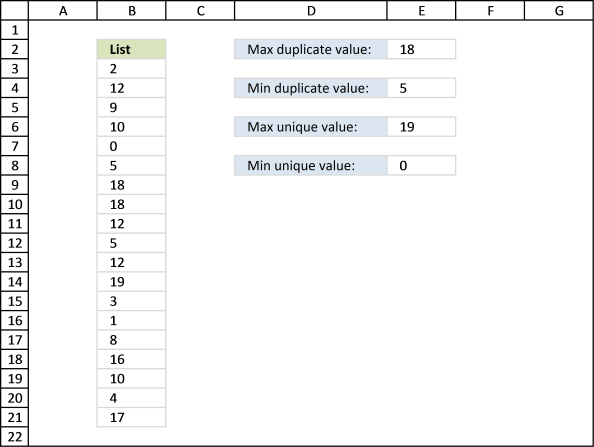Author: Oscar Cronquist Article last updated on November 30, 2018

Question: How do I get the largest and smallest unique and duplicate value?

The image below shows you a list of numbers in column B (\$B\$3:\$B\$21).Max duplicate value, array formula in E2:

The largest number in the list above is 19 but it is a unique number meaning it exists only once. Number 18 is the largest duplicate number, 18 is in cell B9 and B10.

=MAX(IF(COUNTIF(\$B\$3:\$B\$21,\$B\$3:\$B\$21)>1,\$B\$3:\$B\$21,))

To enter an array formula, type the formula in a cell then press and hold CTRL + SHIFT simultaneously, now press Enter once. Release all keys.

The formula bar now shows the formula with a beginning and ending curly bracket telling you that you entered the formula successfully. Don't enter the curly brackets yourself.

### Explaining formula in cell E2

#### Step 1 - Count frequency of each value

The COUNTIF function counts values based on a condition or criteria, in this case, it counts all values in cell range.

COUNTIF(\$B\$3:\$B\$21,\$B\$3:\$B\$21)

becomes

COUNTIF({2; 12; 9; 10; 0; 5; 18; 18; 12; 5; 12; 19; 3; 1; 8; 16; 10; 4; 17},{2; 12; 9; 10; 0; 5; 18; 18; 12; 5; 12; 19; 3; 1; 8; 16; 10; 4; 17})

and returns

{1; 3; 1; 2; 1; 2; 2; 2; 3; 2; 3; 1; 1; 1; 1; 1; 2; 1; 1}.

#### Step 2 - Extract duplicate numbers

The IF function returns the row number if cell is a duplicate. FALSE returns "" (nothing).

IF(COUNTIF(\$B\$3:\$B\$21,\$B\$3:\$B\$21)>1,\$B\$3:\$B\$21,)

becomes

IF({1; 3; 1; 2; 1; 2; 2; 2; 3; 2; 3; 1; 1; 1; 1; 1; 2; 1; 1}>,\$B\$3:\$B\$21,)

becomes

IF({1; 3; 1; 2; 1; 2; 2; 2; 3; 2; 3; 1; 1; 1; 1; 1; 2; 1; 1}>,{2; 12; 9; 10; 0; 5; 18; 18; 12; 5; 12; 19; 3; 1; 8; 16; 10; 4; 17},)

and returns

{0; 12; 0; 10; 0; 5; 18; 18; 12; 5; 12; 0; 0; 0; 0; 0; 10; 0; 0}.

#### Step 3 - Return largest value

The MAX function returns the maximum value from a cell range or array.

MAX(IF(COUNTIF(\$B\$3:\$B\$21,\$B\$3:\$B\$21)>1,\$B\$3:\$B\$21,))

becomes

MAX({0; 12; 0; 10; 0; 5; 18; 18; 12; 5; 12; 0; 0; 0; 0; 0; 10; 0; 0})

and returns 18 in cell E2.Min duplicate value, formula in E4:

The smallest number in the list above is 0 but it is a unique number meaning it exists only once. Number 5 is the smallest duplicate number, 18 is in cell B8 and B12.

=MIN(IF(COUNTIF(\$B\$3:\$B\$21, \$B\$3:\$B\$21)>1, \$B\$3:\$B\$21, ""))

Max unique value, formula in E6:

The largest unique number in the list above is 19.

=MAX(IF(COUNTIF(\$B\$3:\$B\$21, \$B\$3:\$B\$21)=1, \$B\$3:\$B\$21, ))

Min unique value, formula in E8:

The smallest unique number in the list above is 0.

=MIN(IF(COUNTIF(\$B\$3:\$B\$21, \$B\$3:\$B\$21)=1, \$B\$3:\$B\$21, ""))